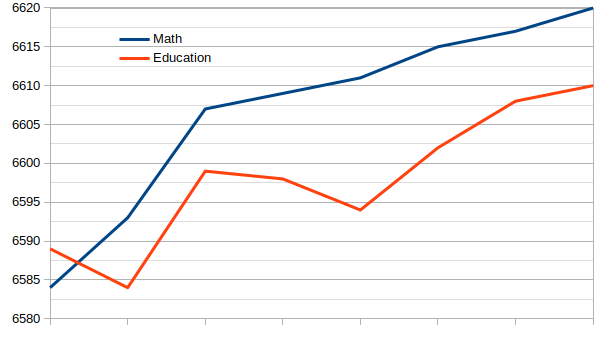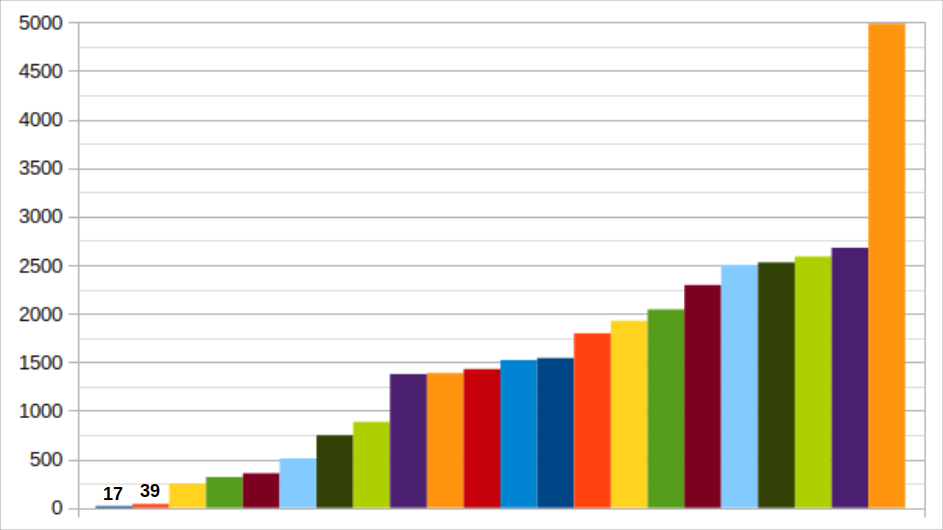# Unicamp fight 2019 – a mathematical analysis

Between the 22nd and 23rd of June 2019, the final of a competition took place to choose the best institute of a large university. The voting period started at 8:00 pm on the 22nd and lasted until 8:00 pm on the 23rd.

The dispute between the Institute of Education and that of Mathematics was fierce, gathered more than 13 thousand votes and ended with a historic and conflicting score. Because between 8:00 pm and 8:00 pm, the score changed several times, either because the vote lasted until the last seconds of the competition, connectivity problems and the updating of votes. This generated conflicts over who should be the “real winner” or whether a draw was just. People posted prints of their screens showing the results at 8:00 pm, but the results were conflicting.

 Time Institute of Education Institute of Mathematics 20h00min 6.589 6.584 6.584 6.593 6.599 6.607 6.598 6.609 6.594 6.611 6.602 6.615 6.608 6.617 6.610 6.620

First point to note, were the “scoreboard-institute” functions growing? That is, whoever voted for an institute does not change or remove their vote? As a matter of logic, the competition itself revolved around making memes and influencing people over which institute to vote for. If everyone already had their votes predefined, the competition would be figurative.

Observing the result in the interval between 20h00min00sec and 20h00min59sec, we can identify (ignoring a possible error caused by the system update), that clearly there were changes or cancellations of votes. In the graph below we place the votes of the Mathematics Institute in an increasing way (remembering that the known scores are paired). Thus, when the Institute of Mathematics had 6.584 votes, the Institute of Education had 6.589 votes. But in the next moment (in relation to the growth of votes of the Institute of Mathematics), when the Institute of Mathematics reached 6.593 votes, the Institute of Education dropped to 6.584 votes.Line graph according to the Mathematics Institute.

Second aspect, the competition had a time limit to count the votes?

From the results of the other stages of this competition, we realized that the difference in votes could not be seen as a problem based on the previous 21 disputes. Because in them, the sharpest result was between the Institute of Computing and the Institute of Economics, with a final difference of 39 votes.

But if we consider the votes of the Institute of Mathematics and of the Institute of Education, its biggest difference in the 20:00 minute (according to the prints) was 17 votes. Less than half of the second worst case, and far from the other differences between the other competition keys, as shown in the graph below:Graph of differences between the disputes of this competition.

Thus, for this atypical case of the competition, it would only be safe to declare a winner if in the entire interval from 20:00 to 00:00 until 20:00 to 59:59 one of the institutes was clearly with the score above the other. We write this as a scoreboard function for each institute (Mat; Edu) in relation to time 0 ≤ t ≤ 59, as follows:

Mat winner if MAX{Edu(t)} < MIN{Mat(t)}.

Edu winner if MAX{Mat(t)} < MIN{Edu(t)}.

 MAX{Edu(t)} ≥ 6610; MIN{Edu(t)} ≤ 6584; MAX{Mat(t)} ≥ 6620; MIN{Mat(t)} ≤ 6584;

With this we arrived that

Mat winner if 6610 ≤ MAX{Edu(t)} < MIN{Mat(t)} ≤ 6584,

so 6610 < 6584 → FALSE!

Edu winner if 6620 ≤ MAX{Mat(t)} < MIN{Edu(t)} ≤ 6584,

so 6620 < 6584 → FALSE!

In this case, the hypothesis that the Institute of Mathematics or Education is a winner is simultaneously false. Thus, we could not infer from this reasoning that one of the two competitors is the winner. Thus justifying the adoption of a tie in the decision of this result, as was also decided in the respective competition but for arguments related to the sending of the simultaneous data referring to the votes.